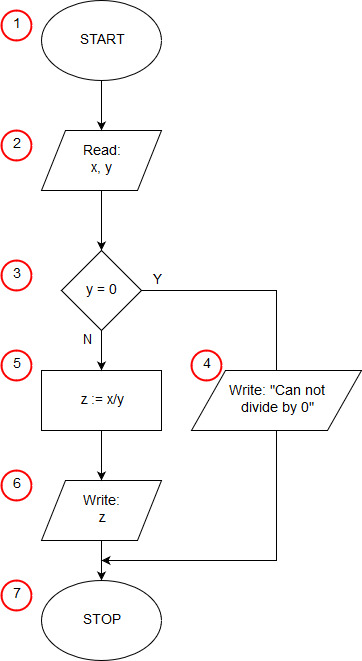# Algorithm of division in C++

<<algorithm#include using namespace std; int main(void){ float x, y; cout<<"x = "; cin>>x; cout<<"y = "; cin>>y; if(y==0) cout<<"Can not divide by 0"; else{ float z; z=x/y; cout<<"z = "<>c; }

In the first line of the program, we include an iostream header file (input/output streams). This is necessary because in the remainder of the program you will want to be able to write on the screen (cout) and load input from the keyboard (cin). In the second line we tell the compiler that we will use std (cin, cout, endl) namespaces in the namespace program. If we did not do this, it would be necessary to use longer qualified names (std::cin, std::cout, std::endl). In C ++, the program starts with the execution of main. In our example, this is the only feature of the program. Line 3 contains the start of the definition of the main function, this is the beginning of our algorithm (block START (1)). In the next line we declare two variables x, y, which we will use in the next moment. In C ++, variables can be declared anywhere before they are used, so we do not immediately declare a variable from here, which may not be needed at all.

Lines 5 through 8 perform an input/output operation (2), where input data is input - dividend and divisible.

The conditional statement of line 9 is a selection block (3) from our algorithm. The instruction checks if y is 0. When the condition is fulfilled, the instruction is written in line 10 (in the input block algorithm (4)). When the condition is not fulfilled, the instruction after the else keyword is executed. In our case, this is a compound statement, enclosed in logical brackets {} between lines 11 and 15. First, in line 12 we declare the variable z, now we know that it will be us. Needed, then in line 13 we compute the quotient of x by y (operation block (5)) and then in line 14 we print the result obtained (input-output block (6)).

The instructions in lines 16-18 have no relation to the implementation of the division algorithm. They stop the program until you enter any character and press enter to prevent the program window from closing immediately.

The curly brace } from line 19 terminates the main function and at the same time runs the entire program (end of the algorithm block (7)).